# Make your Data Talk!

Matplotlib and Seaborn are two of the most powerful and popular data visualization libraries in Python. Read on to learn how to create some of the most frequently used graphs and charts using Matplotlib and Seaborn.comments

By Puneet Grover, Helping Machines Learn.

This article is one of the posts from the `Tackle` category, which can be found on my github repo here.

### Index

1. Introduction
2. Single Distribution Plots (Hist, KDE, -[Box, Violin])
3. Relational Plots (Line, Scatter, Contour, Pair)
4. Categorical Plots(Bar, +[Box, Violin])
5. Multiple Plots
6. Interactive Plots
7. Others
9. References

NOTE:

This post goes along with the Jupyter Notebook available in my Repo on Github: [HowToVisualize]

### 1. Introduction

What is data, nothing but numbers. If we are not visualizing it to get a better understanding of the world inside it, we are missing out on lots of things. I.e. we can make some sense out of data as numbers, but magic happens when you try to visualize it. It makes more sense and it suddenly it becomes more perceivable.

We are sensual beings, we perceive things around us through our senses. Sight, Sound, Smell, Taste and Touch. We can, to some extent, distinguish things around us according to our senses. For data, Sound and Sight seems to be the best options to represent it as it can be easily transformed. And we mostly use Sight as a medium to perceive data because probably we are accustomed to differentiating different object through this sense and also, though in lower level, we are also are accustomed to perceiving things in higher dimensions through this sense which comes in handy in multivariate data sets.

In this post, we look into two of the most popular libraries for visualization of data in Python and use them to make data talk, through visualization:

1.1 Matplotlib

Matplotlib was made keeping MATLAB’s plotting style in mind, though it also has an object oriented interface.

1. MATLAB style interface: You can use it by importing `pyplot` from matplotlib library and use MATLAB like functions.

When using this interface, methods will automatically select current figure and axes to show the plot in. It will be so (i.e. this current figure will be selected again and again for all your method calls) until you use `pyplot.show` method or until you execute your cell in IPython.

2. Object Oriented interface: You can use it like this:

```import matplotlib.pyplot as plt
figure, axes = plt.subplots(2) # for 2 subplots
# Now you can configure your plot by using functions available for these objects.
```

It is low level library and you have total control over your plot.

1.2 Seaborn

Seaborn is a higher level library for visualization, made on top of matplotlib. It is mainly used to make quick and attractive plots without much hassle. Though seaborn tries to give some control over your plots in a fancy way, but still you cannot get everything you desire from it. For that you will have to use matplotlib’s functionality, which you can use with seaborn too (as it is built on matplotlib).

### 2. Distribution PlotsPhoto by Daniel Leone on Unsplash

Distribution plots (or `Probability plots`) tells us how one variable is distributed. It gives us probability of finding a variable in particular range. I.e. if we were to randomly select a number from total range of a variable, it gives us probabilities of this variable being in different ranges.
Distribution plots should be `Normally` distributed, for better results. This is one of the assumptions of all Linear models, i.e. Normality. `Normal distribution` looks like a medium hump on middle with light tails.

Note: If TL;DR (Too Long; Don’t wanna Read), just read initial function used to plot the sub-topic plot and then read through Tips. Eg: here Tip #1 and plt.hist, below.

(Tip #1)

1) You can get away with using `matplotlib.pyplot`'s function's provided parameters for your plots, in most cases. Do look into function's parameters and their description.

2) All `matplotlib`'s functions and even `seaborn`'s functions returns all components of your plot in a dictionary, list or object. From there also you can change any property of your components (in `matplotlib`’s language `Artists`).

Box Plots and Violin Plots are in Categorical Section.

1. Histograms and Kernel Density Estimate Plots (KDEs):
```# Simple hist plot
_ = plt.hist(train_df['target'], bins=5, edgecolors='white')```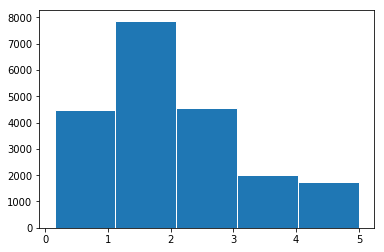```# with seaborn
_ = sns.distplot(train_df['target'])```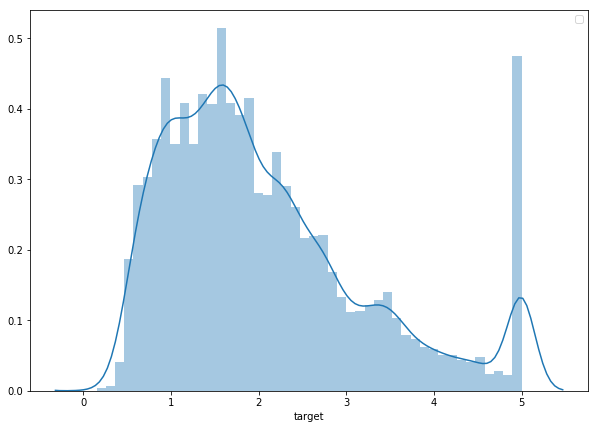(Tip #2)

3) For giving some useful information with your plot or drawing attention to something in plot you can mostly get away with either `plt.text()` or `plt.annotate()`.

4) Most necessary parameter for a plot is‘`label`’, and most necessary methods for a plot are ‘`plt.xlabel`’, ‘`plt.ylabel`’, ‘``` plt.title```’, and ‘`plt.legend`’.

A] To effectively convey your message you should remove all unwanted distractions from your plot like right and top axis, and any other unwanted structure in your plot.

```import matplotlib.pyplot as plt

_ = plt.hist(data, bins=10, color='lightblue',
label=lbl, density=True, ec='white')
plt.legend()
plt.title("Target variable distribution", fontdict={'fontsize': 19,
plt.xlabel("Target Bins")
plt.ylabel("Probability");```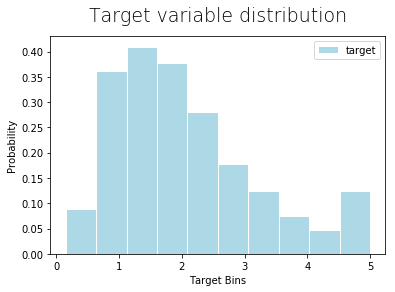Storytelling With Matplotlib (SWMat)

```import matplotlib.pyplot as plt
from SWMat.SWMat import SWMat

swm = SWMat(plt) # Initialize your plot

swm.hist(data, bins=10, highlight=[2, 9])
swm.title("Carefully looking at the dependent variable revealed
some problems that might occur!")

swm.text("Target is a bi-model dependent feature.\nIt
can be <prop fontsize='18' color='blue'> hard to
predict.<\prop>");```

# Thats all! And look at your plot!!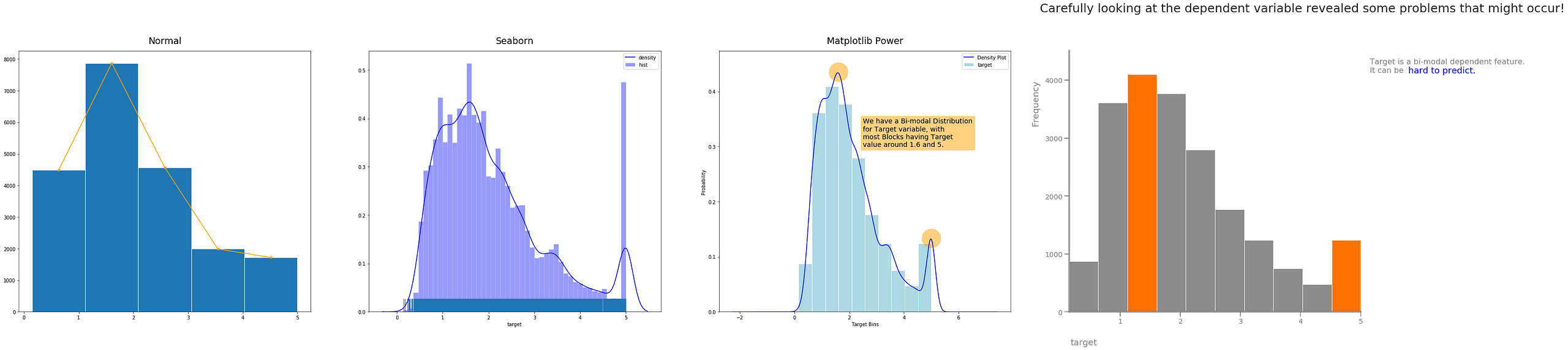1) Normal Matplotlib, 2) Seaborn, 3) Matplotlib Power, 4) Storytelling With Matplotlib

### 3. Relational PlotsRelational plots are very useful in getting relationships between two or more variables. These relationships can help us understand our data more, and probably help us make new variables from existing variables.

This is an important step in `Data Exploration` and `Feature Engineering`.

a) Line Plots:

Line Plots are useful for checking for linear relationship, and even quadratic, exponential and all such relationships, between two variables.

(Tip #3)

5) You can give an aesthetic look to your plot just by using parameters ‘`color`’ / ‘`c`’, ‘`alpha`’ and ‘`edgecolors`’ / ‘`edgecolor`’.

6) `Seaborn` has a parameter ‘`hue`’ in most of its plotting methods, which you can use to show contrast between different classes of a categorical variable in those plots.

B] You should use lighter color for sub parts of plot which you do want in plot but they are not the highlight of the point you want to make.

```plt.plot('AveRooms', 'AveBedrms', data=data,
label="Average Bedrooms")```

plt.legend() # To show label of y-axis variable inside plot
plt.title("Average Rooms vs Average Bedrooms")
plt.xlabel("Avg Rooms ->")
plt.ylabel("Avg BedRooms ->");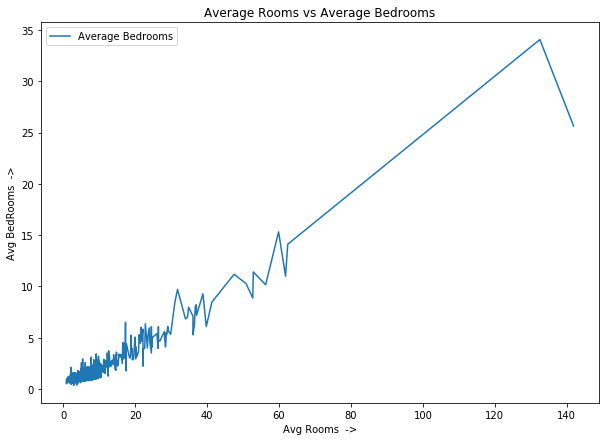You can also color code them manually like this:

```plt.plot('AveRooms', 'AveBedrms', data=data, c='lightgreen')
plt.plot('AveRooms', 'AveBedrms', data=data[(data['AveRooms']>20)],
c='y', alpha=0.7)
plt.plot('AveRooms', 'AveBedrms', data=data[(data['AveRooms']>50)],
c='r', alpha=0.7)```

plt.title("Average Rooms vs Average Bedrooms")
plt.xlabel("Avg Rooms ->")
plt.ylabel("Avg BedRooms ->");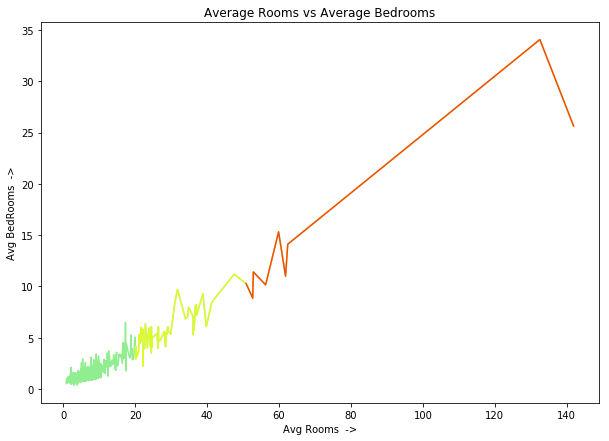```# with seaborn
_ = sns.lineplot(x='AveRooms', y='AveBedrms', data=train_df)```Storytelling With Matplotlib (SWMat)

```import matplotlib.pyplot as plt
from SWMat.SWMat import SWMat
swm = SWMat(plt) # Initialize your plot
swm.hist(data, bins=10, highlight=[2, 9])
swm.title("Carefully looking at the dependent variable revealed
some problems that might occur!")
swm.text("Target is a bi-model dependent feature.\nIt
can be  hard to
predict.<\prop>");
# That's all! And look at your plot!!

```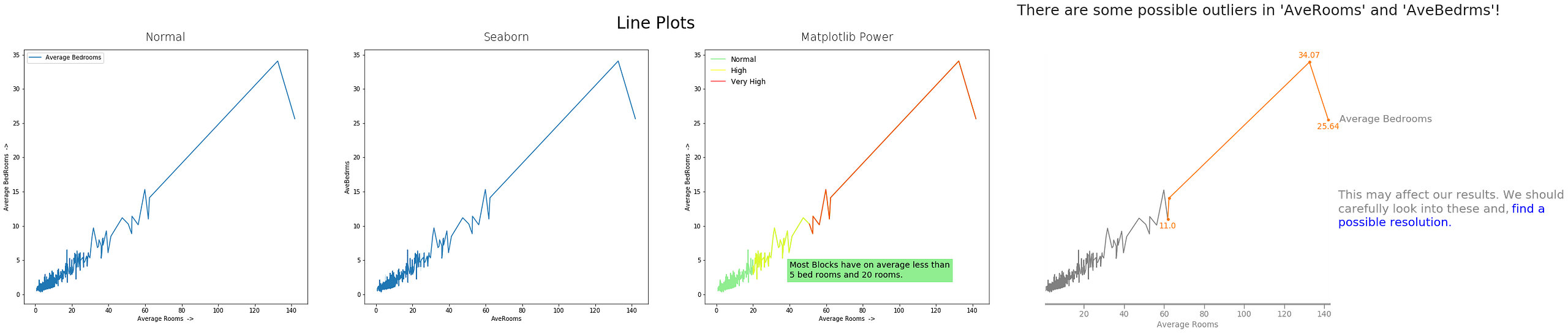1) Normal Matplotlib, 2) Seaborn, 3) Matplotlib Power, 4) Storytelling With Matplotlib

b) Scatter Plots:

Not every relationship between two variables is linear, actually just a few are. These variables too have some random component in it which makes them almost linear, and other cases have a totally different relationship which we would have had hard time displaying with linear plots.

Also, if we have lots of data points, scatter plot can come in handy to check if most data points are concentrated in one region or not, are there any outliers w.r.t. these two or three variables, etc.

We can plot scatter plot for two or three and even four variables if we color code the fourth variable in 3D plot.

(Tip #4)

7) You can set size of your plot(s) in two ways. Either you can import `figure` from `matplotlib` and use method like: ‘`figure(figsize=(width, height))`’ {it will set this figure size for current figure} or you can directly specify `figsize` when using Object Oriented interface like this: `figure, plots = plt.subplots(rows, cols, figsize=(x,y))`.
C] You should be concise and to the point when you are trying to get a message across with data.

```from matplotlib.pyplot import figure
figure(figsize=(10, 7))

plt.scatter('AveRooms', 'AveBedrms', data=data,
edgecolors='w', linewidths=0.1)```

plt.title("Scatter Plot of Average Rooms and Average Bedrooms")
plt.xlabel("Average Bedrooms ->")
plt.ylabel("Average Rooms ->");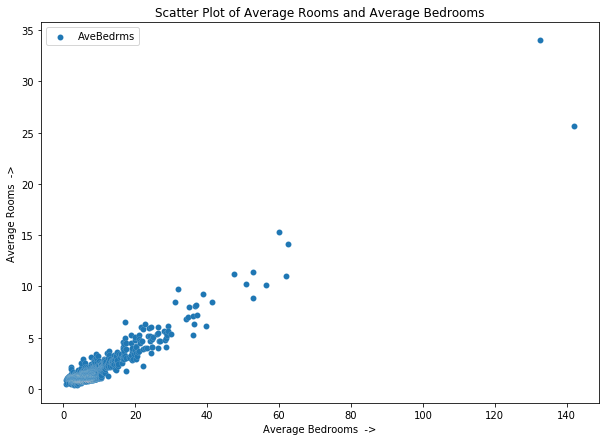```# With Seaborn
from matplotlib.pyplot import figure
figure(figsize=(10, 7))

sns.scatterplot(x='AveRooms', y='AveBedrms', data=train_df,
label="Average Bedrooms");

```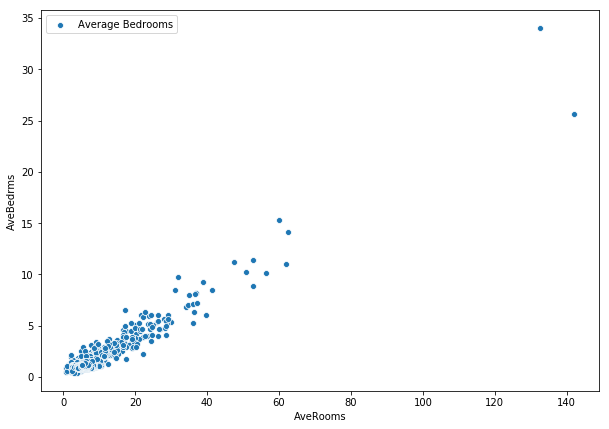By subscribing you accept KDnuggets Privacy Policy

By subscribing you accept KDnuggets Privacy Policy

By subscribing you accept KDnuggets Privacy Policy